Miscellaneous

Practical Geometry
Serial order wise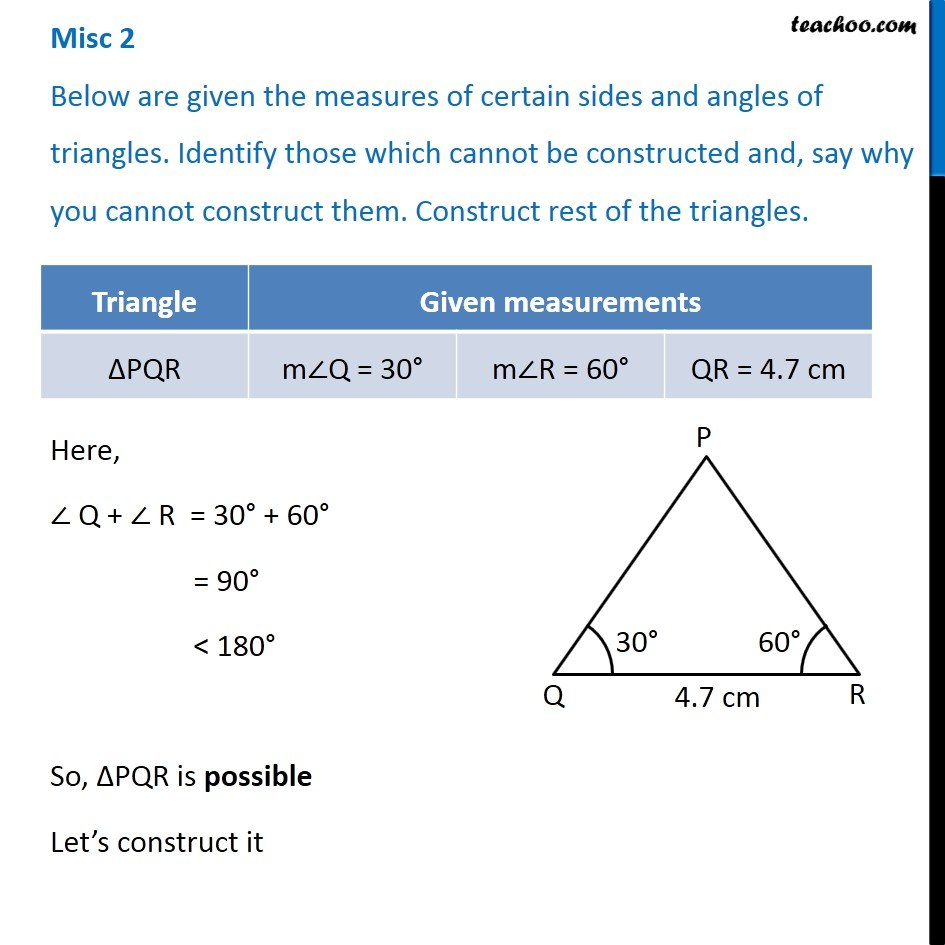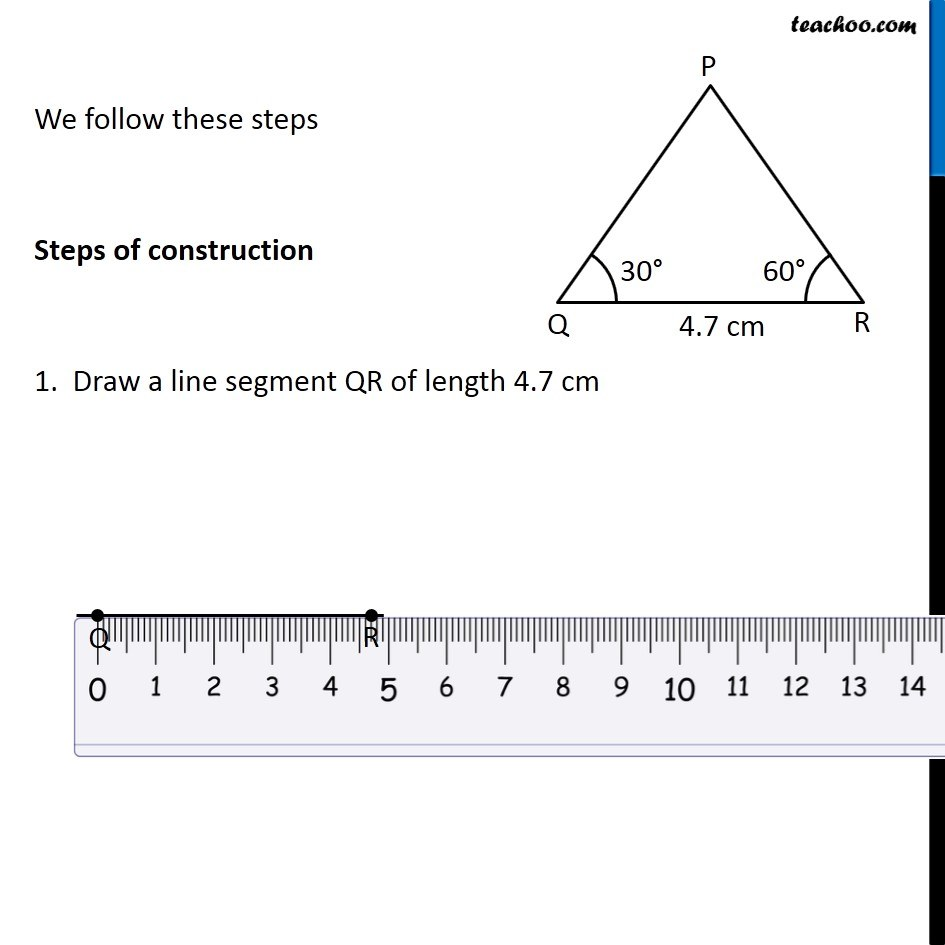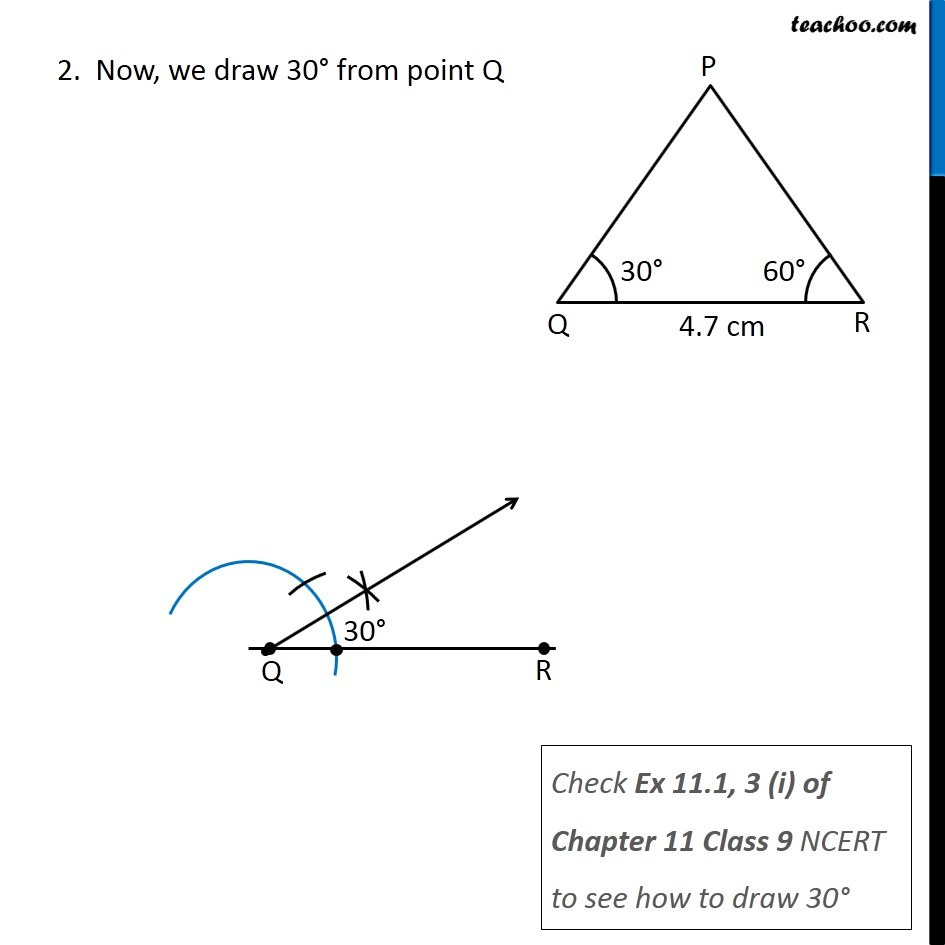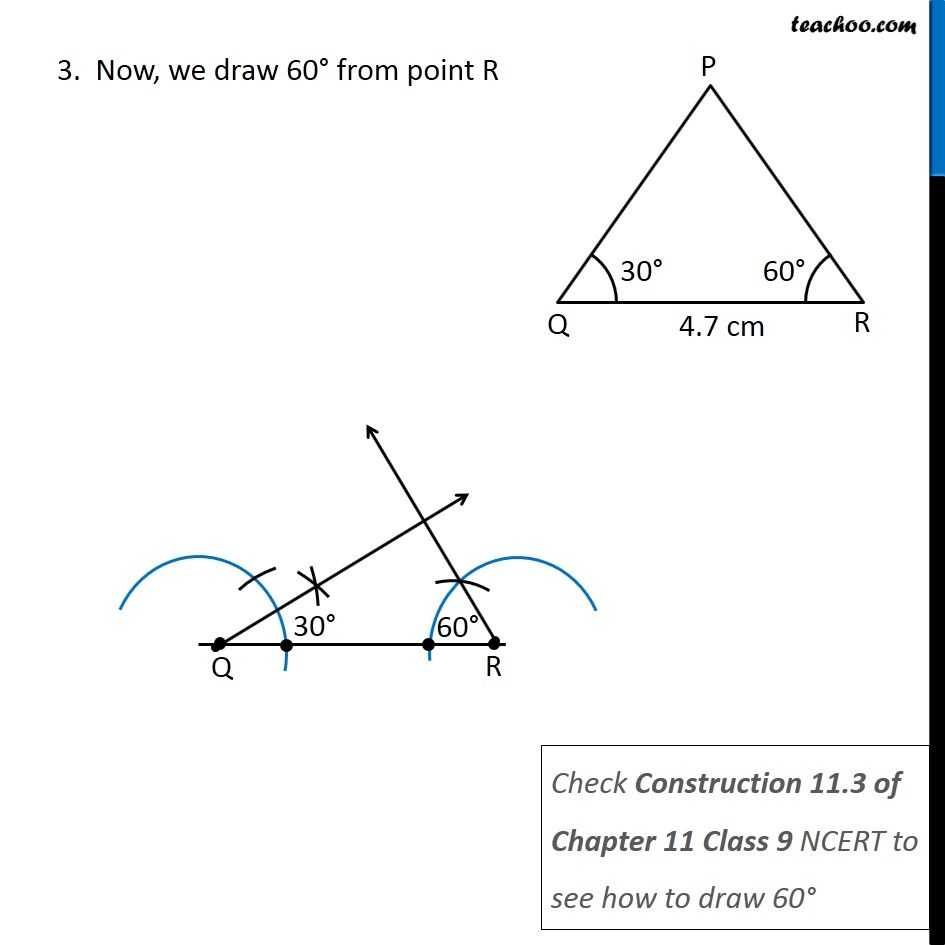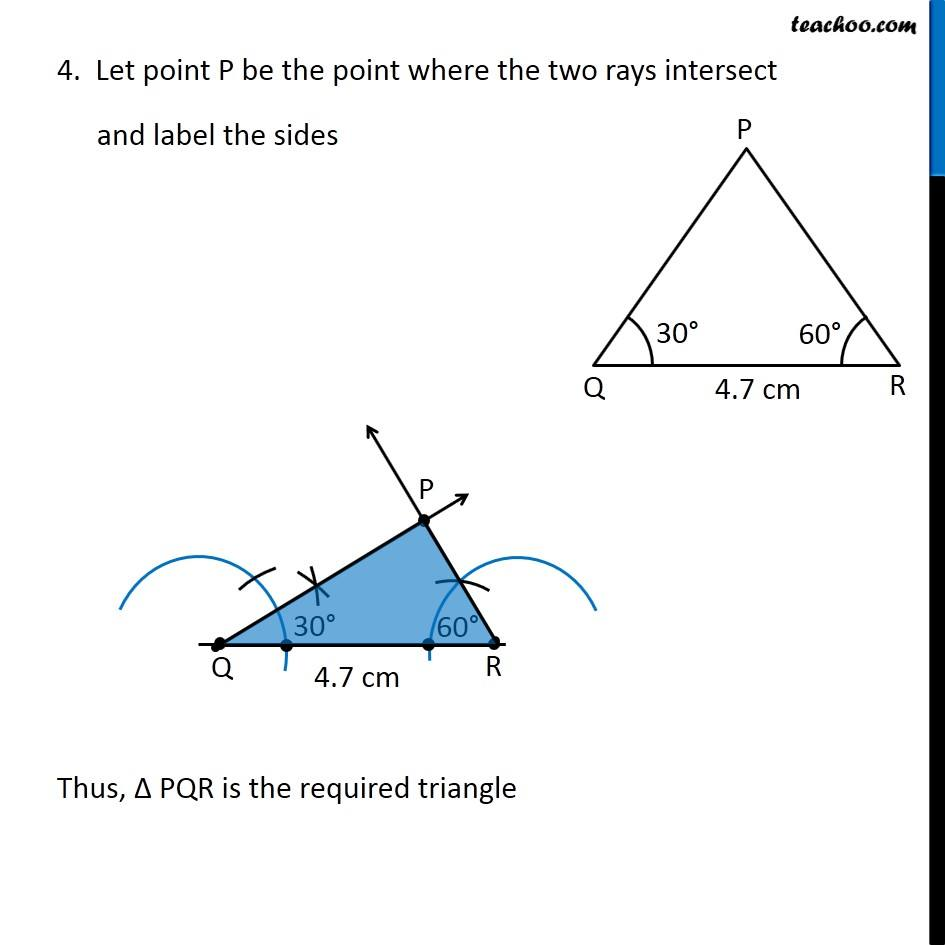Learn in your speed, with individual attention - Teachoo Maths 1-on-1 Class

### Transcript

Misc 2 Below are given the measures of certain sides and angles of triangles. Identify those which cannot be constructed and, say why you cannot construct them. Construct rest of the triangles. Here, ∠ Q + ∠ R = 30° + 60° = 90° < 180° So, ΔPQR is possible Let’s construct it We follow these steps Steps of construction 1. Draw a line segment QR of length 4.7 cm 2. Now, we draw 30° from point Q Check Ex 11.1, 3 (i) of Chapter 11 Class 9 NCERT to see how to draw 30° 3. Now, we draw 60° from point R Check Construction 11.3 of Chapter 11 Class 9 NCERT to see how to draw 60° 4. Let point P be the point where the two rays intersect and label the sides Thus, Δ PQR is the required triangle